UPSC  >  Exercise 12.2 NCERT Solutions - Heron’s Formula

# Exercise 12.2 NCERT Solutions - Heron’s Formula - NCERT Textbooks (Class 6 to Class 12) - UPSC

## Application of Heron's Formulas in Finding Areas of Quadrilaterals

Many fields are in the shape of quadrilaterals. Similarly, various surfaces are polygons. To find their areas, we divide the surface into triangular parts and then use Heron’s formula for finding areas of the triangles.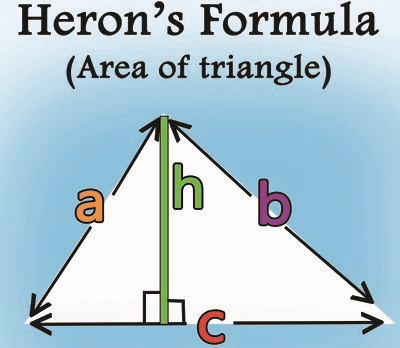## Exercise 12.2

Q1. A park, in the shape of a quadrilateral ABCD, has – C = 90°, AB = 9 m, BC = 12 m, CD = 5 m and AD = 8 m. How much area does it occupy?
Ans:
Let us join B and D, such that ΔBCD is a right-angled triangle.
We have area of ΔBCD = (1/2)x base x altitude
=(1/2)x 12 x 5 m2
= 30 m2
Now, to find the area of ΔABD, we need the length of BD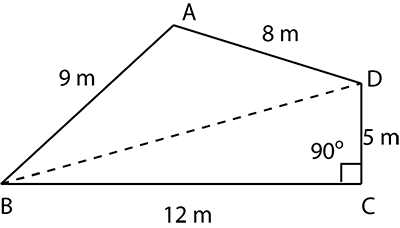∴ In right ΔBCD, BD2 = BC+ CD2 [Pythagoras theorem]
⇒ BD2 = 12+ 52
⇒ BD2 = 144 + 25 = 169 = 13
⇒ BD =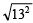= 13 m
Now, for ΔABD, we have a = 9 m, b = 8 m, c = 13 m
∵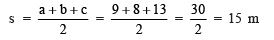∴ Area of ΔABD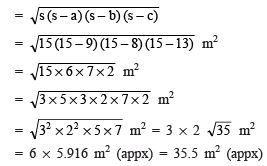∴ Area of quadrilateral ABCD = area of ΔBCD + area of ΔABD
= 30 m+ 35.5 m2 = 65.5 m2 (appx)

Q2. Find the area of a quadrilateral ABCD in which AB = 3 cm, BC = 4 cm, CD = 4 cm, DA = 5 cm and AC = 5 cm.
Ans:
For ΔABC,

a= 3 cm, b= 4cm and c = 5 cm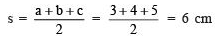∴ Area of  ΔABC,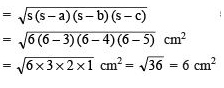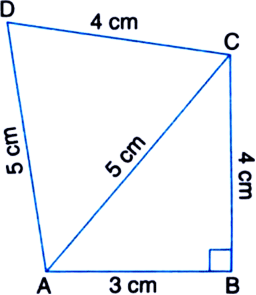For ΔACD,

a=5 cm, b = 4cm and c= 5cm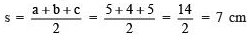For ΔACD,

a=5 cm, b = 4cm and c= 5cm∴ Area of ΔACD,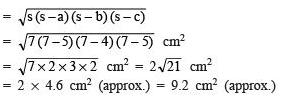Now, area of quadrilateral ABCD = area of ΔABC + area of ΔACD
= 6 cm2 + 9.2 cm2 (approx.)
= 15.2 cm(approx.)

Q3. Radha made a picture of an aeroplane with coloured paper as shown in Fig. Find the total area of the paper used.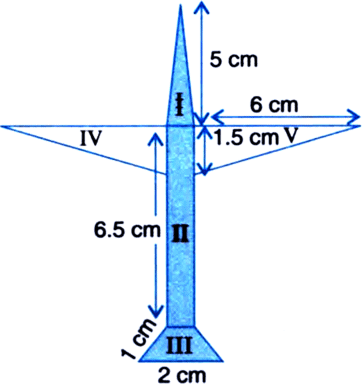Ans: Area of surface I It is an isosceles triangle whose sides are a = 5 cm, b = 5 cm, c = 1 cm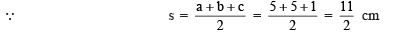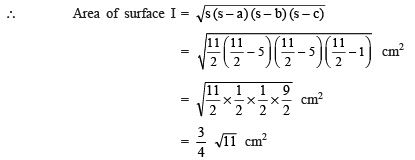= 0.75 x 3.3 cm2 (approx.) = 2.475 cm(approx.)

Area of surface II It is a rectangle with length of 6.5 cm and breadth of 1 cm.
∴ Area of rectangle II = Length x Breadth
= 6.5 x 1 = 6.5 cm
Area of surface III It is a trapezium whose parallel sides are 1 cm and 2 cm as shown in the adjoining figure. Its height is given by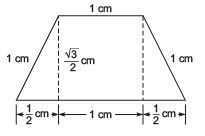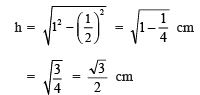Note: The perpendicular distance between the parallel sides is called the height of the trapezium.

∵ Area of a trapezium = 1/2(sum of the parallel sides) x Height

∴ Area of trapezium III =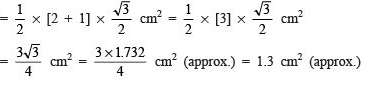Area of surface IV It is a right triangle with base as 6 cm and height as 1.5 cm.
∴ Area of right triangle IV =(1/2)x base x height
=(1/2)x 6 x 1.5 cm2 = 4.5 cm2
Area of surface V
∵ Right triangle V ≌  Right triangle IV
∴ Area of right triangle V = Area of right triangle IV = 4.5 cm
Thus, the total area of the paper used = (area I) + (area II) + (area III) + (area IV) + (area V)
= [2.475 cm2 (approx.)] + [6.5 cm2] + [1.3 cm2 (approx.)] + [4.5 cm2] + [4.5 cm2]
= 19.275 cm= 19.3 cm2 (approx.)

Q4. A triangle and a parallelogram have the same base and the same area. If the sides of the triangle are 26 cm, 28 cm and 30 cm, and the parallelogram stands on the base 28 cm, find the height of the parallelogram.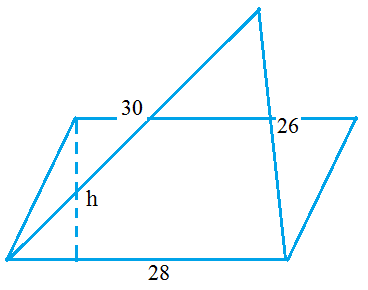Ans: For the given triangle, we have a = 28 cm, b = 30 cm, c = 26 cm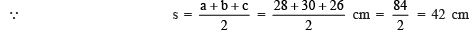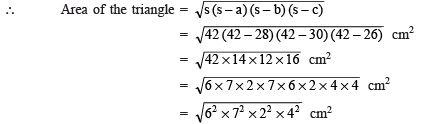= 6 x 7 x 2 x 4 cm= 336 cm2
∵ [Area of the given parallelogram] = [Area of the given triangle]
∴ [Area of the parallelogram] = 336 cm
∴ [Base of the parallelogram] x [Height of the parallelogram] = 336 cm
⇒ [28 cm] x [h cm] = 336 cm2

⇒ h= (336/28)cm = 12
Thus, the required height of the parallelogram = 12 cm

Q5. A rhombus shaped field has green grass for 18 cows to graze. If each side of the rhombus is 30 m and its longer diagonal is 48 m, how much area of grass field will each cow be getting?
Ans:
Here, each side of the rhombus = 30 m
One of the diagonal = 48 m
Since a diagonal divides the rhombus into two congruent triangles.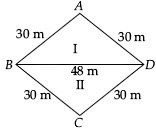Sides of first triangle are a = 30 m, b = 30 m, c = 48 m

∵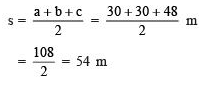∴ Area of triangle I =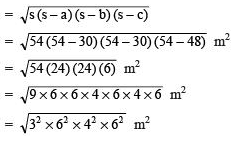= 3 x 6 x 4 x 6 m2 = 432 m2
∴ Area of second triangle = 432 m2
⇒ Total area of both triangles = 432 cm2 + 432 m2  = 864 m2
⇒ The area of the rhombus = 864 m2
Thus, area of grass for 18 cows = 864 m
⇒ Area of grass for 1 cow = (864/18)m2 = 48 m2

Q6. An umbrella is made by stitching 10 triangular pieces of cloth of two different colours (see figure), each piece measuring 20 cm, 50 cm and 50 cm. How much cloth of each colour is required for the umbrella?
Ans:
Sides of each triangular piece are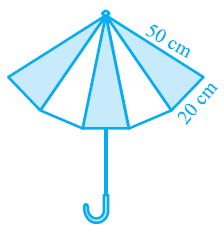a = 20 cm, b = 50 cm, c = 50 cm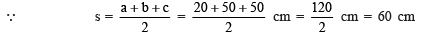∴ Area of each triangular piece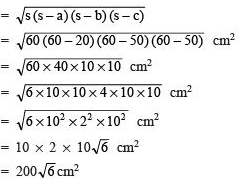⇒ Area of 5 triangular pieces of one colour = 5 x 200/√6cm= 1000/√6 cm2 Area of 5 triangular pieces of other colour = 1000/√6 cm2

Note: Total triangular pieces are 10, i.e. five triangular pieces for each colour.

Q7. A kite in the shape of a square with a diagonal 32 cm and an isosceles triangle of base 8 cm and sides 6 cm each is to be made of three different shades as shown in the figure. How much paper of each shade has been used in it?
Ans:
Area of triangle I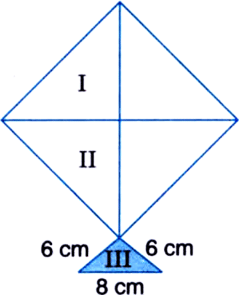Diagonal = 32 cm
∴ Height of triangle =(1/2)(32) cm= 16 cm
∵ Area of triangle = (1/2)x base x height
∴ Area of triangle I= (1/2)x 32 x 16 cm2 = 256 cm2
Area of triangle II ∵ Diagonal of a square divides it into two congruent triangles.
∴ Area of triangle II = Area of triangle I
⇒ Area of triangle II = 256 cm2

Area of triangle III The triangle at the base is having sides as a = 8 cm, b = 6 cm, c = 6 cm
∵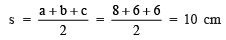∴ Area of triangle III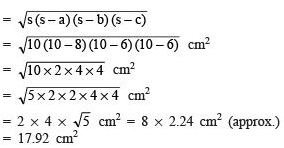Thus, the area of different shades are: Area of shade I = 256 cm2,
Area of shade II = 256 cm2
Area of shade III = 17.92 cm2

Q8. A floral design on a floor is made up of 16 tiles which are triangular, the sides of the triangle being 9 cm, 28 cm and 35 cm (see figure). Find the cost of polishing the tiles at the rate of 50p per cm2.
Ans:
There are 16 equal triangular tiles.
Area of a triangle Sides of the triangle are a = 9 cm, b = 28 cm, c = 35 cm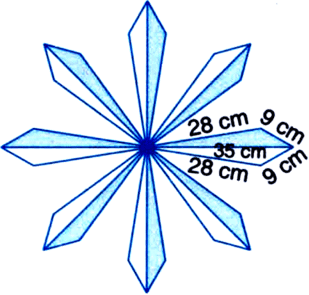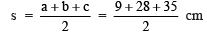=(72/2)cm = 36 cm

∴  Area of the triangle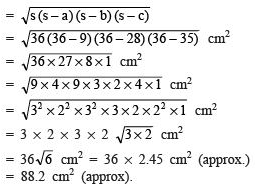Area of 16 triangles: Total area of all the triangles = 16 x 88.2 cm2 (approx.) = 1411.2 cm2 (approx.) Cost of polishing the tiles Rate of polishing = ₹ 0.5 per cm
∴ Cost of polishing all the tiles = ₹ 0.5 x 1411.2
= ₹(5/10) x (14112/10)= ₹705.60 (approx.)

Q9. A field is in the shape of a trapezium whose parallel sides are 25 m and 10 m. The non-parallel sides are 14 m and 13 m. Find the area of the field.
Ans:
The given field is in the form of a trapezium ABCD such that parallel sides AB = 10 m and DC = 25 m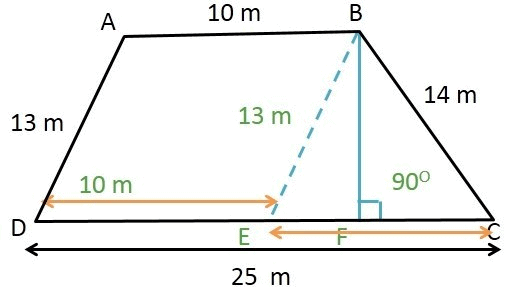Non-parallel sides are 13 cm and 14 cm.
We draw BE || AD, such that BE = 13 cm.
The given field is divided into two shapes:

(i) parallelogram ABED (ii) DBCE.
Area of ΔBCE: Sides of the triangle are a = 13 m, b = 14 m, c = 15 m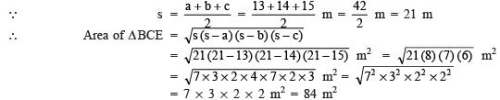Let the height of the ΔBCE corresponding to the side 15 m be ‘h’ metres
∵ Area of a triangle = (1/2)x base x height
∴  (1/2)x 15 x h= 84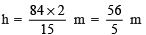x m = (56/5)m
Area of parallelogram ABED
∵ Area of a parallelogram = base x height
∴ Area of parallelogram ABED = 10 x (1/2)m= 2 x 56 m2 = 112 m2
Thus, area of the field = Area of ΔBCE + Area of parallelogram ABED
= 84 m2 + 112 m2 = 196 m2

The document Exercise 12.2 NCERT Solutions - Heron’s Formula | NCERT Textbooks (Class 6 to Class 12) - UPSC is a part of the UPSC Course NCERT Textbooks (Class 6 to Class 12).
All you need of UPSC at this link: UPSC

## FAQs on Exercise 12.2 NCERT Solutions - Heron’s Formula - NCERT Textbooks (Class 6 to Class 12) - UPSC

 1. Can Heron's Formula be used to find the area of any quadrilateral?Ans. No, Heron's Formula can only be used to find the area of a quadrilateral if it is a cyclic quadrilateral, meaning that all four vertices lie on a circle.
 2. How do you determine if a quadrilateral is cyclic?Ans. A quadrilateral is cyclic if the sum of opposite angles is equal to 180 degrees. In other words, if the opposite angles are supplementary, then the quadrilateral is cyclic.
 3. Can Heron's Formula be used to find the area of a rectangle or square?Ans. No, Heron's Formula is not applicable to rectangles or squares because they are not cyclic quadrilaterals. The area of a rectangle or square can be found using simpler formulas specific to those shapes.
 4. Are there any other formulas that can be used to find the area of non-cyclic quadrilaterals?Ans. Yes, there are other formulas available to find the area of non-cyclic quadrilaterals. One commonly used formula is the Brahmagupta's Formula, which takes into account the lengths of the sides and the lengths of the diagonals.
 5. How is Heron's Formula derived for finding the area of a triangle?Ans. Heron's Formula is derived by using the semi-perimeter of a triangle and the lengths of its sides. By substituting the values into the formula, the area of the triangle can be calculated.

## NCERT Textbooks (Class 6 to Class 12)

1404 docs|679 tests

## NCERT Textbooks (Class 6 to Class 12)

1404 docs|679 tests

### How to Prepare for UPSC

Read our guide to prepare for UPSC which is created by Toppers & the best Teachers
Signup to see your scores go up within 7 days! Learn & Practice with 1000+ FREE Notes, Videos & Tests.
10M+ students study on EduRev
Track your progress, build streaks, highlight & save important lessons and more!(Scan QR code)
Related Searches

,

,

,

,

,

,

,

,

,

,

,

,

,

,

,

,

,

,

,

,

,

;